# A Frustratingly Easy Approach for Entity and Relation Extraction

## PURE

PURE(the Princeton University Relation Extraction system)简单的分为实体模型关系模型两部分, 训练时完全分开训练. 因为是Pipeline, 所以关系模型使用实体的Golden Label训练.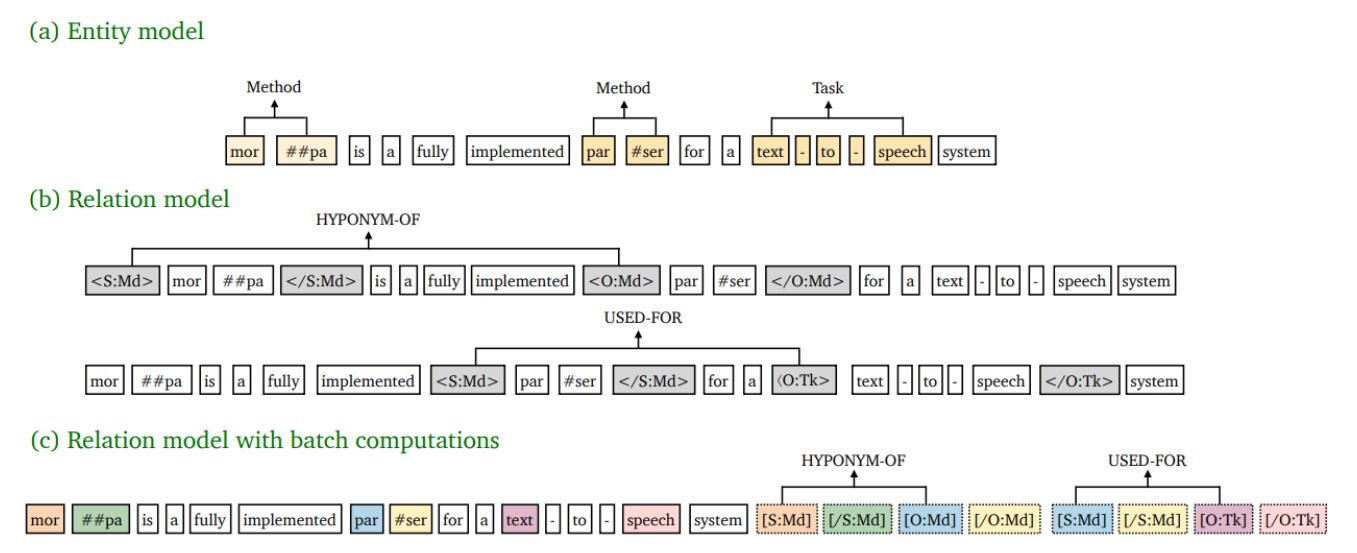• (a) 实体模型: 根据Span的表示, 判断给定的Span的实体类型.
• (b) 关系模型: 根据Span对的表示, 判断给定的Span对的关系类型.
• (c) 批计算的关系模型: 关系模型的一种加速实现.

### Problem Definition

• NER: 对于预定义的实体类型集合$\mathcal{E}$, 基于Span的NER任务为判断每个Span $s_i \in S$ 的实体类型$y_e(s_i) \in \mathcal{E}$, 若Span不是实体, 则记为$y_e(s_i) = \epsilon$. 任务的最终输出为$Y_e=\set{(s_i, e): s_i \in S, e \in \mathcal{E}}$.
• RE: 对于预定义的关系类型集合$\mathcal{R}$, RE任务为判断每个Span对$s_i\in S, s_j \in S$ 之间的关系类型$y_r(s_i, s_j) \in \mathcal{R}$, 若Span对之间不存在关系, 则记为$y_r(s_i, s_j) = \epsilon$. 任务最终输出为$Y_r = \set{(s_i, s_j, r): s_i, s_j \in S, r \in \mathcal{R}}$.

### Entity Model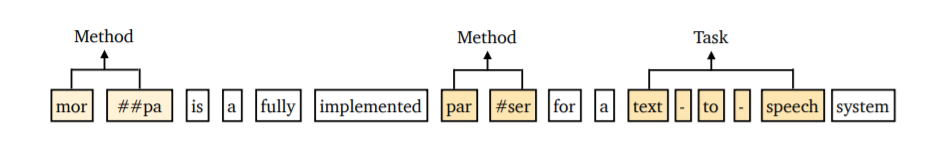$$\mathbf{h}_{e}\left(s_{i}\right)=\left[\mathbf{x}_{\mathrm{START}(i)} ; \mathbf{x}_{\mathrm{END}(i)} ; \phi\left(s_{i}\right)\right]$$

Span的表示仅简单的使用了Span边界以及Span长度信息, 而非把Span内部所有信息全部囊括. 基于Span的模型经常只使用边界上的信息, 可能是假设边界蕴含的语义更强.

$$P_{e}\left(e \mid s_{i}\right)=\operatorname{softmax}\left(\mathbf{W}_{e} \mathrm{FFNN}\left(\mathbf{h}_{e}\left(s_{i}\right)\right)\right.$$

### Relation Model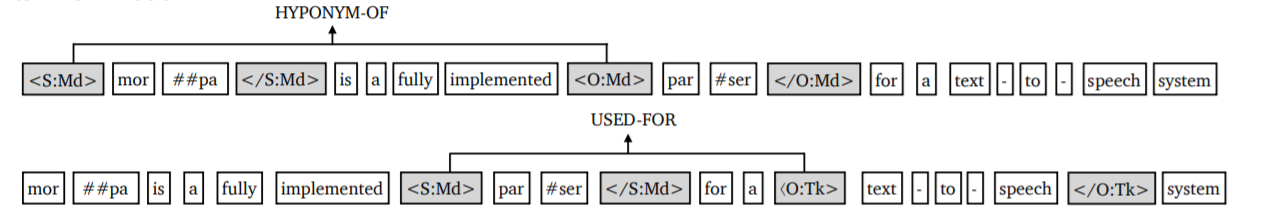\begin{aligned} &\widehat{X}=\ldots\left\langle\operatorname{S}: e_{i}\right\rangle, x_{\operatorname{START}(i)}, \ldots, x_{\operatorname{END}(i)},\left\langle/ \mathrm{S}: e_{i}\right\rangle \\ &\ldots\left\langle\mathrm{O}: e_{j}\right\rangle, x_{\mathrm{START}(j)}, \ldots, x_{\mathrm{END}(j)},\left\langle/ \mathrm{O}: e_{j}\right\rangle, \ldots \end{aligned}

$$\mathbf{h}_{r}\left(s_{i},s_{j}\right)=\left[\widehat{\mathbf{x}}_{\widehat{\operatorname{START}}(i)} ; \widehat{\mathbf{x}}_{\widehat{\operatorname{START}}(j)}\right]$$

$\widehat{\operatorname{START}}(i), \widehat{\operatorname{START}}(j)$ 为$\left\langle\mathrm{S}: e_{i}\right\rangle, \left\langle\mathrm{O}: e_{j}\right\rangle$ 的在$\widehat{X}$ 的下标.

$$P_{r}\left(r \mid s_{i}, s_{j}\right)=\operatorname{softmax}\left(\mathbf{W}_{r} \mathbf{h}_{r}\left(s_{i}, s_{j}\right)\right)$$

### Training & inference

\begin{aligned} &\mathcal{L}_{e}=-\sum_{s_{i} \in S} \log P_{e}\left(e_{i}^{\ast} \mid s_{i}\right) \\ &\mathcal{L}_{r}=-\sum_{s_{i}, s_{j} \in S_{G}, s_{i} \neq s_{j}} \log P_{r}\left(r_{i, j}^{\ast} \mid s_{i}, s_{j}\right) \end{aligned}

$e_i^\ast$ 为$s_i$ 的真实实体类型, $r_{i, j}^\ast$ 为$s_i, s_j$ 间的真实关系类型.

### Differences from DYGIE++

1. 作者用两个独立的Encoder分别做NER和RE任务, 而非用MTL(Multi - task Learning)的角度去看待.并且关系模型所需的实体类型信息能完全产生于实体模型.
2. 引入了Text Marker, 在关系模型中Span对的表示不同.
3. 使用跨句信息, 而不是使用Beam Search和图网络.

### Efficient Batch Computations

\begin{aligned} &\mathrm{P}\left(\left\langle\mathrm{S}: e_{i}\right\rangle\right), \mathrm{P}\left(\left\langle/ \mathrm{S}: e_{i}\right\rangle\right):=\mathrm{P}\left(x_{\mathrm{START}(i)}\right), \mathrm{P}\left(x_{\mathrm{END}(i)}\right) \\ &\mathrm{P}\left(\left\langle\mathrm{O}: e_{j}\right\rangle\right), \mathrm{P}\left(\left\langle/ \mathrm{O}: e_{j}\right\rangle\right):=\mathrm{P}\left(x_{\mathrm{START}(j)}\right), \mathrm{P}\left(x_{\mathrm{END}(j)}\right) \end{aligned}

$P(\cdot)$ 代表取Token的位置ID.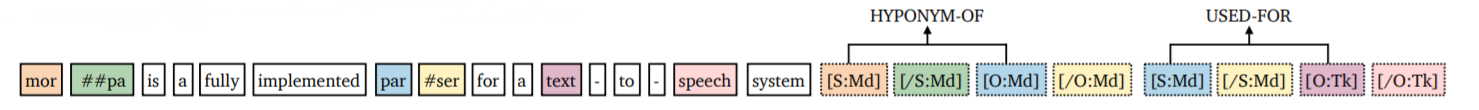## Experiments

### Datasets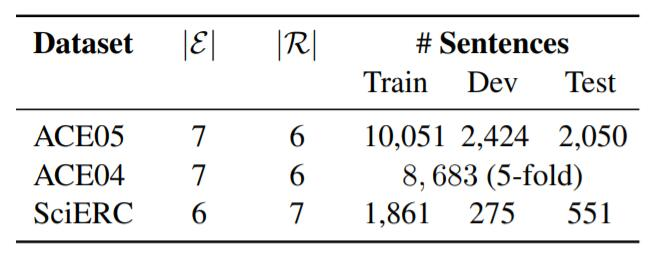### Main Results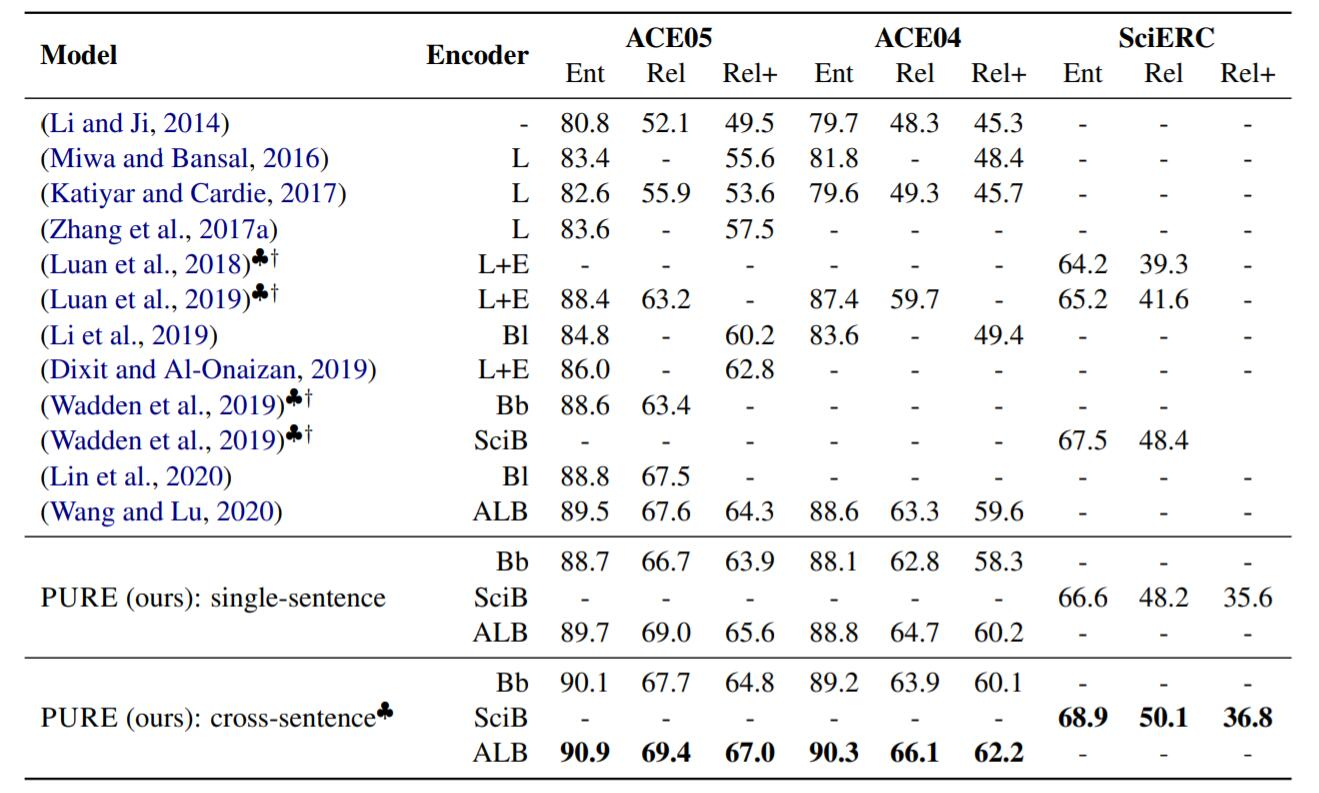♣代表引入跨句, †代表引入额外数据. 结果中L = LSTM, L + E = LSTM + Elmo, Bb = BERT - base, Bl = BERT - large, SciB = SciBERT(规模与Bert - base相同), ALB = ALBERT - xxlarge - v1. Rel为实体边界准确且关系准确, Rel+为实体边界和实体类型, 关系均准确.

### Batch Computations and Speedup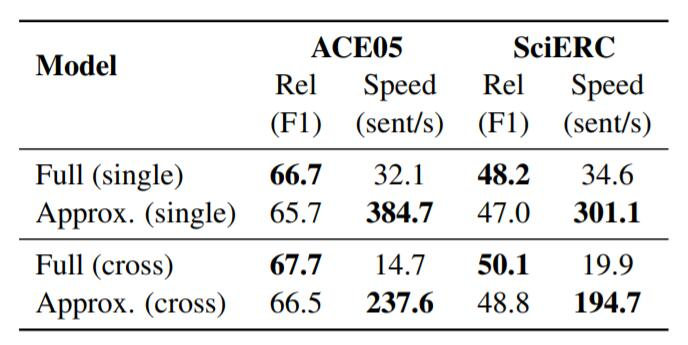### Analysis

#### Importance of Typed Text Markers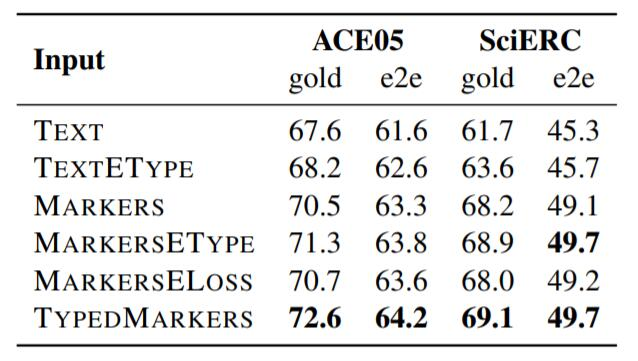gold为使用Golden Entity, e2e代表端到端, 也就是训练时直接使用实体模型的结果.

• TEXT: 直接用原Text的每个实体的的起始Token Embedding.
• TEXTETYPE: 在TEXT基础上拼接Entity Type Embedding.
• MARKERS: 使用不包含实体类型的边界Text Marker的Embedding.
• MARKERSETYPE: 在MARKERS基础上拼接实体类型Embedding.
• MARKERSELOSS: 使用不包含实体类型的边界Text Marker Embedding, 并引入一个辅助Loss, 用边界表示判断实体类型.
• TYPEDMARKERS: 正文中所使用的方法.

#### Modeling Entity-Relation Interactions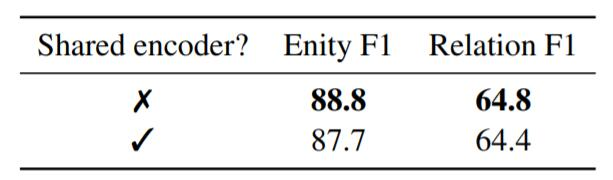#### Mitigating Error Propagation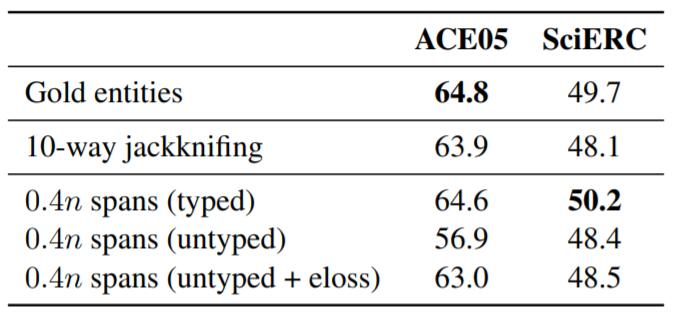10 - way jackkniﬁng就是十折交叉, 即为把数据划分成十份, 并且每个模型都用其中9份训练, 预测最后1份, 每个模型都对应着1份的预测结果, 把它们作为关系模型的输入, 然后训练关系模型.

#### Effect of Cross - sentence Context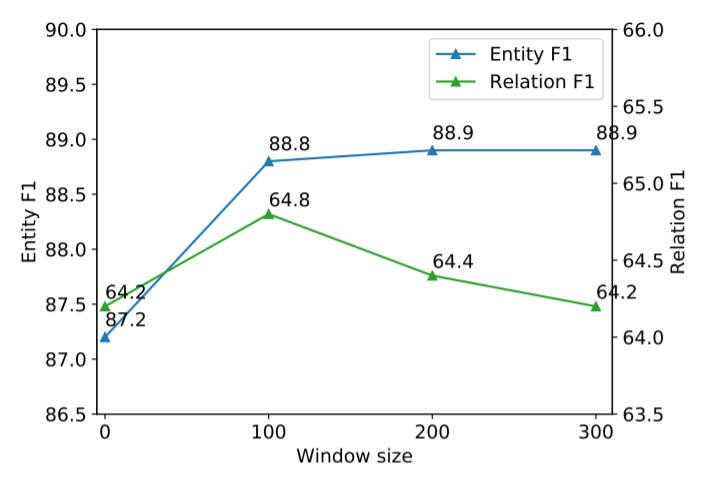$W=100$ 后提升不大, 甚至对关系模型有负面影响.

## Summary

上一篇PFN: A Partition Filter Network for Joint Entity and Relation Extraction

2022-01-12

目录